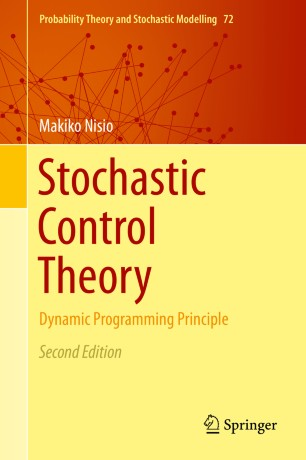# Stochastic Control Theory

## Dynamic Programming Principle

• Makiko NisioBook

Part of the Probability Theory and Stochastic Modelling book series (PTSM, volume 72)

1. Front Matter
Pages i-xv
2. Makiko Nisio
Pages 1-30
3. Makiko Nisio
Pages 31-78
4. Makiko Nisio
Pages 79-115
5. Makiko Nisio
Pages 117-151
6. Makiko Nisio
Pages 153-207
7. Makiko Nisio
Pages 209-244
8. Back Matter
Pages 245-250

### Introduction

This book offers a systematic introduction to the optimal stochastic control theory via the dynamic programming principle, which is a powerful tool to analyze control problems.

First we consider completely observable control problems with finite horizons. Using a time discretization we construct a nonlinear semigroup related to the dynamic programming principle (DPP), whose generator provides the Hamilton–Jacobi–Bellman (HJB) equation, and we characterize the value function via the nonlinear semigroup, besides the viscosity solution theory. When we control not only the dynamics of a system but also the terminal time of its evolution, control-stopping problems arise. This problem is treated in the same frameworks, via the nonlinear semigroup. Its results are applicable to the American option price problem.

Zero-sum two-player time-homogeneous stochastic differential games and viscosity solutions of the Isaacs equations arising from such games are studied via a nonlinear semigroup related to DPP (the min-max principle, to be precise). Using semi-discretization arguments, we construct the nonlinear semigroups whose generators provide lower and upper Isaacs equations.

Concerning partially observable control problems, we refer to stochastic parabolic equations driven by colored Wiener noises, in particular, the Zakai equation. The existence and uniqueness of solutions and regularities as well as Itô's formula are stated. A control problem for the Zakai equations has a nonlinear semigroup whose generator provides the HJB equation on a Banach space. The value function turns out to be a unique viscosity solution for the HJB equation under mild conditions.

This edition provides a more generalized treatment of the topic than does the earlier book Lectures on Stochastic Control Theory (ISI Lecture Notes 9), where time-homogeneous cases are dealt with. Here, for finite time-horizon control problems, DPP was formulated as a one-parameter nonlinear semigroup, whose generator provides the HJB equation, by using a time-discretization method. The semigroup corresponds to the value function and is characterized as the envelope of Markovian transition semigroups of responses for constant control processes. Besides finite time-horizon controls, the book discusses control-stopping problems in the same frameworks.

### Keywords

dynamic programming principle nonlinear semigroup stochastic differential game stochastic optimal control viscosity solution

#### Authors and affiliations

• Makiko Nisio
• 1
1. 1.Kobe UniversityKobeJapan